# Python 语法之列表

• 2022 年 9 月 22 日
四川
• 本文字数：5495 字

阅读完需：约 18 分钟

eight_immortal = ['汉钟离', '张果老', '韩湘子', '铁拐李', '吕洞宾', '何仙姑', '蓝采和', '曹国舅']print(eight_immortal)

eight_immortal 就是一个列表，[ ]包含的每一个信息称为元素，'汉钟离'、'张果老'、'韩湘子'等就是元素。在 Python 中，列表有如下特性：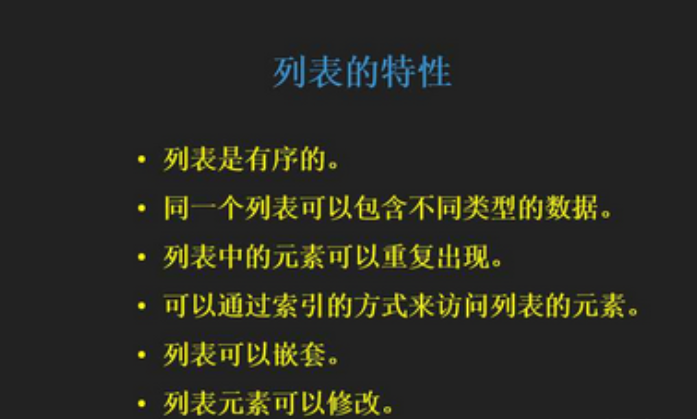# 1.有序

eight_immortal1 = ['汉钟离', '张果老', '韩湘子', '铁拐李', '吕洞宾', '何仙姑', '蓝采和', '曹国舅']eight_immortal2 = ['吕洞宾', '何仙姑', '蓝采和', '曹国舅', '汉钟离', '张果老', '韩湘子', '铁拐李']print(eight_immortal1 == eight_immortal2)

# 2.元素的数据类型

eight_immortal = ['汉钟离', 1, '张果老', 2, '韩湘子', 3, '铁拐李', 4,'吕洞宾', 5, '何仙姑', 6, '蓝采和', 7, '曹国舅', 8]print(eight_immortal)

# 3.元素可以重复

eight_immortal1 = ['铁拐李', '铁拐李', '何仙姑', '何仙姑', '蓝采和', '蓝采和']print(eight_immortal1)

# 4.元素的访问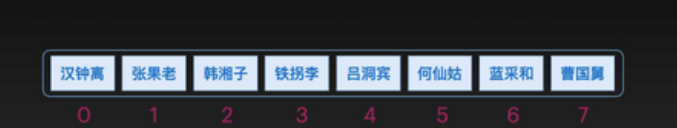eight_immortal = ['汉钟离', '张果老', '韩湘子', '铁拐李', '吕洞宾', '何仙姑', '蓝采和', '曹国舅']print(eight_immortal)

eight_immortal = ['汉钟离', '张果老', '韩湘子', '铁拐李', '吕洞宾', '何仙姑', '蓝采和', '曹国舅']print(eight_immortal)print(eight_immortal)print(eight_immortal)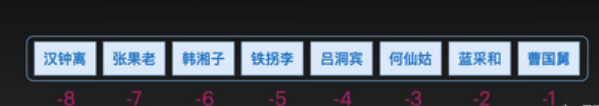eight_immortal = ['汉钟离', '张果老', '韩湘子', '铁拐李', '吕洞宾', '何仙姑', '蓝采和', '曹国舅']print(eight_immortal[-5])

eight_immortal = ['汉钟离', '张果老', '韩湘子', '铁拐李', '吕洞宾', '何仙姑', '蓝采和', '曹国舅']print(eight_immortal[-8])print(eight_immortal[-4])print(eight_immortal[-1])

eight_immortal = ['汉钟离', '张果老', '韩湘子', '铁拐李', '吕洞宾', '何仙姑', '蓝采和', '曹国舅']print(eight_immortal[3:6])print(eight_immortal[2:])print(eight_immortal[:5])print(eight_immortal[:])

eight_immortal = ['汉钟离', '张果老', '韩湘子', '铁拐李', '吕洞宾', '何仙姑', '蓝采和', '曹国舅']print(eight_immortal[-5:-2])print(eight_immortal[-5:])print(eight_immortal[:-2])print(eight_immortal[:])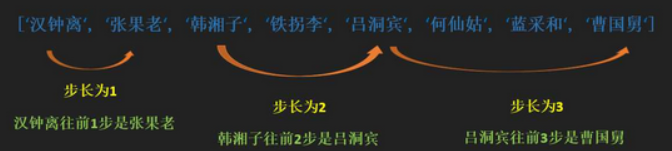eight_immortal = ['汉钟离', '张果老', '韩湘子', '铁拐李', '吕洞宾', '何仙姑', '蓝采和', '曹国舅']print(eight_immortal[0:7:2])

# 5.列表可以嵌套

my_list = ['a', ['bb', ['ccc', 'ddd'], 'ee', 'ff'], 'g', ['hh', 'ii'], 'j']print(my_list)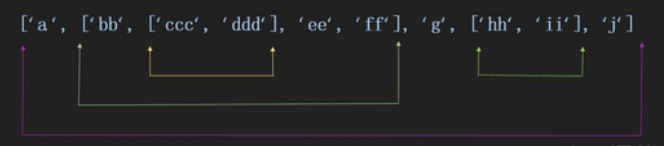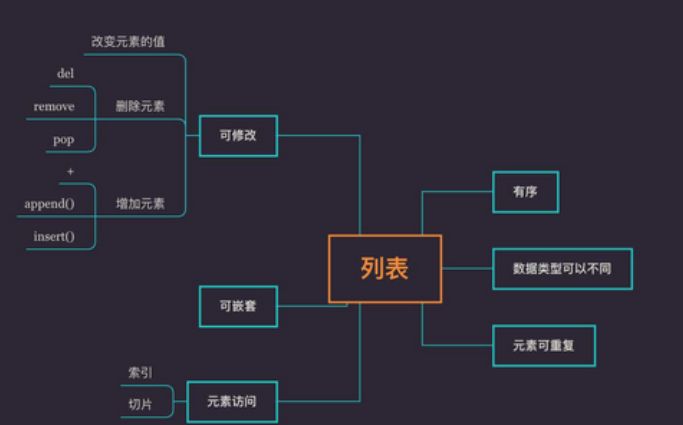my_list = ['a', ['bb', ['ccc', 'ddd'], 'ee', 'ff'], 'g', ['hh', 'ii'], 'j']print(my_list)print(my_list)print(my_list)print(my_list)

# 6.列表元素可以修改

### 6.1 改变元素的值

#### 6.1.1 一次改变一个值

eight_immortal = ['汉钟离', '张果老', '韩湘子', '铁拐李', '吕洞宾', '何仙姑', '蓝采和', '曹国舅']eight_immortal = '逍遥闲散'eight_immortal = '清婉动人'print(eight_immortal)

#### 6.1.2 一次改变多个值

eight_immortal = ['汉钟离', '张果老', '韩湘子', '铁拐李', '吕洞宾', '何仙姑', '蓝采和', '曹国舅']eight_immortal[0:3] = ['逍遥闲散', '倒骑毛驴','巧夺造化']

eight_immortal = ['汉钟离', '张果老', '韩湘子', '铁拐李', '吕洞宾', '何仙姑', '蓝采和', '曹国舅']eight_immortal[0:3] = ['逍遥闲散', '倒骑毛驴','巧夺造化']print(eight_immortal)

eight_immortal = ['汉钟离', '张果老', '韩湘子', '铁拐李', '吕洞宾', '何仙姑', '蓝采和', '曹国舅']eight_immortal[0:3] = [10, 20, 30]eight_immortal[-5:-1] = [40, 50, 60, 70]print(eight_immortal)

### 6.2 删除元素

del 列表名[]，铁拐李在列表中的位置为 eight_immortal，因此删除语句为 del eight_immortal

eight_immortal = ['汉钟离', '张果老', '韩湘子', '铁拐李', '吕洞宾', '何仙姑', '蓝采和', '曹国舅']del eight_immortalprint(eight_immortal)

eight_immortal = ['汉钟离', '张果老', '韩湘子', '铁拐李', '吕洞宾', '何仙姑', '蓝采和', '曹国舅']del eight_immortal[0:3]print(eight_immortal)

### 6.3 使用+往列表中增加元素

eight_immortal = ['汉钟离', '张果老', '韩湘子', '铁拐李', '吕洞宾', '何仙姑', '蓝采和', '曹国舅']print(eight_immortal)eight_immortal += ['容成公', '李耳', '、董仲舒','张道陵','严君平','李八百','范长生','尔朱先生']print(eight_immortal)

### 6.4 改变列表的方法

#### 6.4.1 append()

eight_immortal = ['汉钟离', '张果老', '韩湘子', '铁拐李']print(eight_immortal)eight_immortal.append('吕洞宾')print(eight_immortal)eight_immortal.append(['何仙姑', '蓝采和', '曹国舅'])print(eight_immortal)

#### 6.4.2 insert()

eight_immortal = ['汉钟离', '张果老', '韩湘子', '铁拐李']print(eight_immortal)eight_immortal.insert(3, '吕洞宾')print(eight_immortal)eight_immortal.insert(4, ['何仙姑', '蓝采和', '曹国舅'])print(eight_immortal)

#### 6.4.3 remove()

eight_immortal = ['汉钟离', '张果老', '韩湘子', '铁拐李', '吕洞宾', '何仙姑', '蓝采和', '曹国舅']print(eight_immortal)eight_immortal.remove('汉钟离')print(eight_immortal)eight_immortal.remove('骆宾王')

#### 6.4.4 pop(index)

eight_immortal = ['汉钟离', '张果老']print(eight_immortal)eight_immortal.pop()print(eight_immortal)

eight_immortal = ['汉钟离', '张果老', '韩湘子', '铁拐李', '吕洞宾', '何仙姑', '蓝采和', '曹国舅']print(eight_immortal)eight_immortal.pop(1)print(eight_immortal)

pop()传入的参数为索引值，而不是具体的元素值。pop()的返回值为删除的元素。

eight_immortal = ['汉钟离', '张果老', '韩湘子', '铁拐李', '吕洞宾', '何仙姑', '蓝采和', '曹国舅']name = eight_immortal.pop(1)print(name)### 向阳逐梦

InfoQ签约作者、阿里云“乘风者计划”签约博主

## 评论